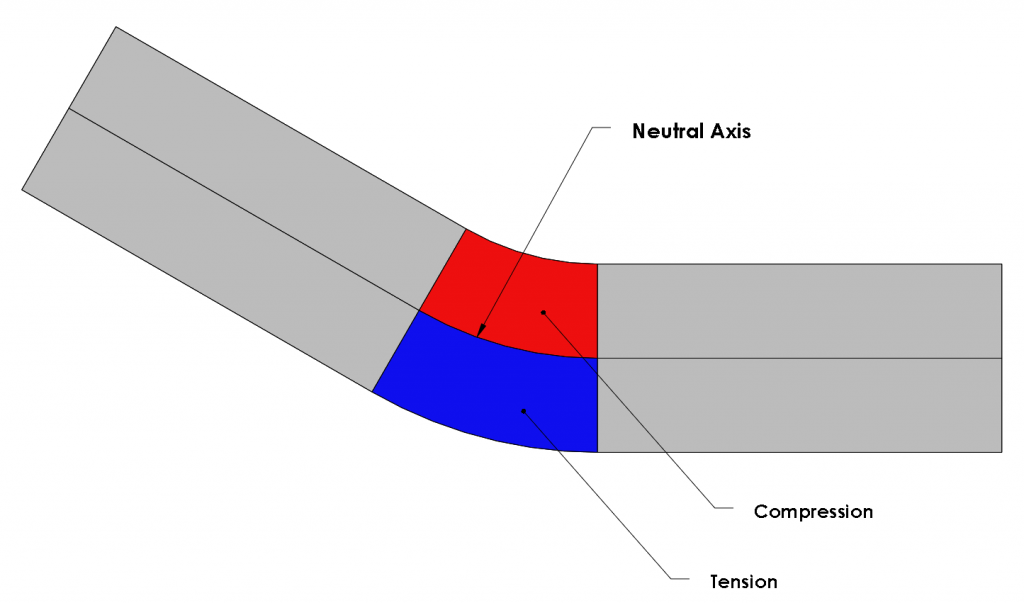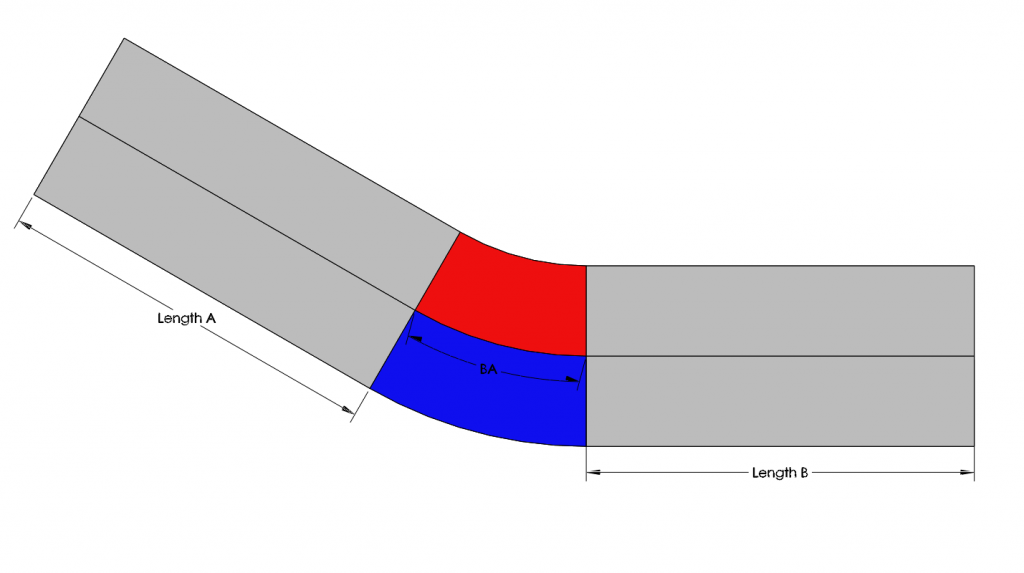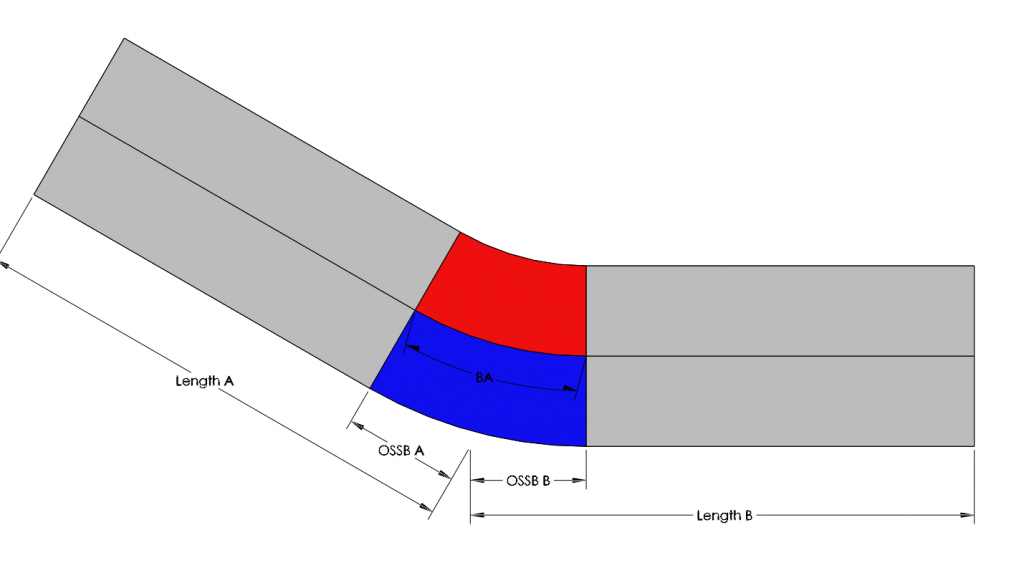When I look at the many SOLIDWORKS sheet metal bend parameter options, I feel a little like Goldilocks stumbling into the bear’s cottage. I have all these options and it’s hard to decide which one is, “just…right.” To make this decision, you need to communicate with the manufacturer of your sheet metal parts and know your options. In this post, I am going to discuss the bend parameter options to help you make these decisions.First, I want to establish why and what bend parameters are. When a piece of sheet metal is bent, the material length will change. This change is represented by the bend parameters and needs to be considered when working with sheet metal so that the appropriate flat pattern is used to get the desired end results. For this reason the bend parameters are only implemented into your part when it is flattened.Secondly, I would like to discuss the theoretical calculations, which can be used to calculate the length of the bend. Once we have a full understanding of how to calculate a flat pattern, we can then pick and choose how we want to apply this information through the bend parameters. So here comes the math! I promise it won’t be that bad…

The bent area will be divided by a neutral axis. On one side of the neutral axis, the area will be in compression, and, on the opposite side, the area will be in tension. The length of the neutral axis will represent the length of the bend when flattened. Here is how we calculate the length of the neutral axis.S = r x θ

S = Arc Length

r = Radius from the Neutral Axis

θ = Angle Defined by Bend (In Radians!)r = rbend + t

r = Radius for Neutral Axis

t = Position of Neutral Axis

Therefore:

S =( rbend + t ) x θ

Ideally, the two forces will be equal and opposite so that the neutral axis lies centered in the material thickness. However, this is not always the case. The neutral axis may shift because of the compression and tension on the part due to the bend, and this needs to be taken into account. A ratio of the neutral axis relative to the thickness is applied to represent this and is called the K-Factor.

K = t / T

K = K-Factor

t = Position of Neutral Axis

T = Thickness of Part

The K-Factor equation, solved for t, can be substituted for the position of the neutral axis from the previous equation. As a result the implemented equation for length of the neutral axis now looks like this:

S =( rbend + KT ) x θ

The length of the neutral axis can now be applied to the lengths of the unbent sections of the sheet to find the overall length required by the flat pattern. Depending on how the lengths of the unbent sections are measured, there are two different equations that can be used.

One is called bend allowance, when the lengths are measured to the edge of the bend and are added to the bend allowance. The bend allowance is calculated by using the length of the neutral axis.Ltotal = LengthA + LengthB + BA

LengthA = Length of Flat

LengthB = Length of Flat

BA = Bend Allowance

The second is called bend deduction, when the lengths are measured to the virtual sharp of the bend and the bend deduction is subtracted. The bend deduction is calculated by subtracting the bend allowance from the outside setback lengths of the bend.Ltotal = LengthA + LengthB – BD

Ltotal = Length of Flat Pattern

LengthA = Length of Flat

LengthB = Length of Flat

BD = Bend Deduction, where

BD = OSSBA+OSSBB – BA

OSSBA = Outside Setback

OSSBB = Outside Setback

BA = Bend Allowance

Now how does this information translate into SOLIDWORKS? In SOLIDWORKS, you will see five options for bend parameters: bend table, gauge table, K-Factor, bend allowance, bend deduction, and bend calculation table.A bend or gauge table can be created to represent every radius and angle combination you will want to use. It can be filled with values calculated using the bend allowance or bend deduction equations above or with exact measured values off the machines. These tables yield the most precise results.

However, what if you are just trying to get a rough idea or visual representation? There is no need to create a detailed table for every scenario in this case. To accommodate a rough estimate, SOLIDWORKS has given you the opportunity to apply one K-Factor, bend allowance, or bend deduction value for all bend scenarios. If that is too broad, you can use the bend calculation option to assign values to a range of angles and radii.

Whether you are looking for a bend parameter that is “too small,” “too big,” or “just right,” SOLIDWORKS has an option for you to produce a flat pattern of your sheet metal part that will be the best fit for your needs.